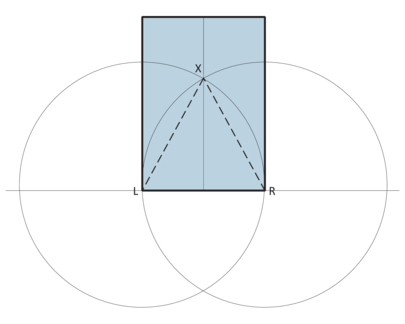# 3. Why does the “60 degree fold” work?

Part 2 analysed the silver rectangle: a rectangle that makes two rectangles that have the same proportions. We used similarity and proportion to prove that ratio of the sides of are . A bronze rectangle () divides into three rectangles of the same proportion. Any rectangle can be divided into four rectangles of the same proportion using the midpoints of each side.

Any triangle can be divided into four triangles of the same proportion using the midpoints of each side. Did you find the silver triangle and bronze triangle? How far could you generalise?Figure 3-1 S Silver and bronze triangles (left and centre) are closely related to silver and bronze rectangles. A triangle that divides into five similar triangles has lengths 1, 2 and sqrt(5) (right).

The model for this article is the Cube From Thirds. It uses two kinds of trisection: dividing a length into thirds, and dividing a right angle into three equal angles. (It has been proved that it is impossible to trisect an arbitrary angle with an unmarked straight edge and pair of compasses. However, using the same tools, it is possible to trisect a straight line segment – some naive mathematicians believed they could then trisect an angle, but a little thought reveals why trisecting lengths and angles are different.)

Unlike earlier articles, here I start with something “known to be true” and then try to prove it: trisecting a right angle by folding. Fold the lower right corner to the centre line and at the same time make the fold go through the lower left corner.Figure 3-2 A rectangle with a 60 degree fold (left). Proving the 60 degree fold (right).

As suggested in the first article, let’s add some extra lines to help us. Repeat the 60 degree fold on the right hand side. The symmetry now shows us that there’s an equilateral triangle because the base length from L to R is the same as L to X and R to X.

Here is a proof by analogy. Children in school geometry are taught a number of constructions using a pair of compasses (circle drawer). First, draw a straight line. Set the pair of compasses to a fixed length. Draw a circle centred on one point on the line. Now draw a circle of the same size centred on the intersection of the line and first circle. The two circles meet at a point that is equidistant from the two circle centres. This is the same as the point X made by folding.Figure 3-3 Construction of an equilateral triangle using a straight edge and a pair of compasses

The 60 degree fold can be generalised in at least two ways: one way is to trisect angles less than 90 degrees, the other is to divide the edge length in special ways. The latter is the subject of the next part. In the meantime, how can you use the 60 degree fold to divide an edge into three equal parts? What other methods are possible?